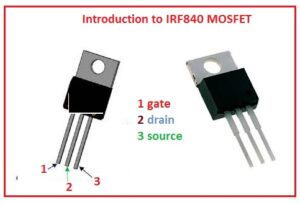Hello friends i hope you all are doing great. In this post, I am going to explain about IRF840 MOSFET. This category of MOSFET is used in such an application where high speed and high voltage is needed since it provides these features and with that, it has less value of on-state resistance. It belongs to the N channel MOSFET family. As this is N channel so the movement of the current or conduction process is done by the free electrons.

In this post we will cover each and every parameter such as pinout, features circuit, and some other parameter related to this module so let’s get started with an introduction to IRF840 MOSFET.

#### Introduction to IRF840 MOSFET

• The IRF840 is an N-channel power metal oxide field effect transistor or MOSFET that can used for switching the process of load value to the five volts.
• It has the ability to do the switching process for a load that uses eight amperes and for turning on it the threshold voltage value is ten volts at the gate and source pinout.
• As it is used for a high-speed switch circuit that has a large value of voltage and current so the voltage value about its gate terminal is large and so it interlinking with the input or output pinout of the central processing unit is not possible.
• For such an application where less value of gate current is needed than used the IRF540N.
• This module has three pinout that are drain gate and source.
• the one and main drawback of this MOSFET is that it offers a large value of RDS that is 0.85 ohm.
• Due to this cause it not preferred for such application where large switching efficacy is needed.
• There is a need of drive circuitry to supply ten volts to the gate pinout of MOSFET for that circuitry construction used the transistors.
•  The price of these devices is very less and has less value of thermal resistance and it used in DC to DC convert circuitry.
• If we do a comparison between BJT and MOSFET we come to know that the BJT is a current control device and MOSFET is a voltage control device.#### Pinout of IRF840 MOSFET

• There is three main pinouts of this component drain, gate, and source which are described here.
• Source: This terminal is used to provide current elimination from the module and value of current for extreme is eight amperes.
• Gate: It used to regulate the biasing process of the MOSFET for this threshold voltage value is ten volts.
• Drain: This pinout is used to provide current to the MOSFET#### Features of IRF840 MOSFET

• The main features of this model are described here with detail.
• It belongs to the N channel family of MOSFET.
• The value of drain to source voltage used for breakdown is five hundred volts.
• The value of the drain current for this MOSFET is eight amperes.
• It exists in the To-220 packaging.
• The value of the gate threshold voltage is ten volts.
• The value of rise time is twenty-three nS and the fall time is twenty Neno seconds.
• The value of drain to source resistance is 0.85 ohms.

### RF840 MOSFET Modes of Operation

• There are three modess of operation it has cutoff, linear, and saturation.

Cutoff mode:

• If the voltage applied at gate is less than the threshold voltage, MOSFET is operating in cutoff mode. In this mode of operation there is no channel creation between the source and drain and zero current flow through MOSFET

Linear mode:

• If the voltage applied is larger than the threshold voltage and less than the saturation voltage, this MOSFET is in a linear mode of operation. In this mode MOSFET operates like the variable resistance and the curent flowing through the device is proportional to the voltage applied at the gate terminal.

Saturation mode:

• If the voltage given at the gate is larger than the saturation voltage MOSFET is in saturation mode. Here channel between the source and drain is created and MOSFET operates like closed switch and large amount of curent flowing

#### Application of IRF840

• The most common application is described here.
• It used in such a circuit that used for speed control of the motor.
• It is the main part of the inverted circuitry..
• It used in light-emitting diode dimmers.
• For such applications where high-speed switching is needed.
• For conversion of Dc to DC converter it used.

So friends that is a detailed post about IRF840. In this post, I tried to cover each and every fact of this MOSFET. If you have any further query ask in the comments thanks for reading have a nice da